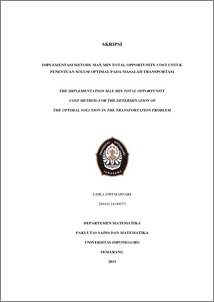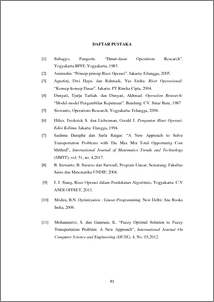# RELATION BETWEEN MINIMUM VERTEX COVER AND MINIMUM EDGE COVER ON COMPLETE FLOWER GRAPH WITH INDEPENDENCE NUMBER, 1-FACTOR, AND CRITICAL FACTOR

Africhati, Maulida Dwi (2019) RELATION BETWEEN MINIMUM VERTEX COVER AND MINIMUM EDGE COVER ON COMPLETE FLOWER GRAPH WITH INDEPENDENCE NUMBER, 1-FACTOR, AND CRITICAL FACTOR. Undergraduate thesis, UNDIP.Preview
PDF
46KbPDF
Restricted to Repository staff only

582KbPreview
PDF
45Kb

## Abstract

Let G be a connected graph. Vertex cover is defined by A V G  ( ) such that every edge in graph G is incident to at least one vertex in A . Edges cover is defined by B E G  ( ) such that every vertex in graph G is incident to at least one edge in B . Complete flower graph is defined as tensor product from two cycle graphs C C m n  for m  2 and n  2 which is denoted by mxn f . This paper discusses the minimum vertex cover and the minimum edge cover on complete flower graph based on their relationship with independence number, 1-factor and critical factor. Minimum vertex cover is equal to minimum edge cover if and only if the independence number is equal to maximum matching of complete flower graph. Minimum vertex cover is equal to independence number and is equal to half of vertex number if and only if complete flower graph have 1-factor. Minimum vertex cover is equal to half of vertex number if and only if complete flower graph is critical factor

Item Type: Thesis (Undergraduate) Q Science > QA Mathematics Faculty of Science and Mathematics > Department of Mathematics 84196 INVALID USER 11 Jun 2022 05:58 11 Jun 2022 05:58

Repository Staff Only: item control page